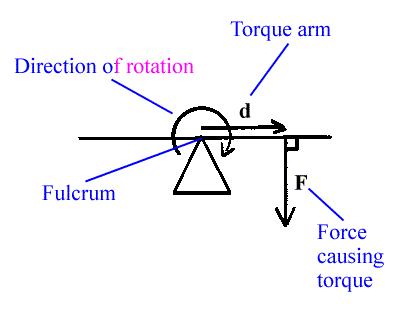# define torque with explanation and some exp...AKASH GOYAL AskiitiansExpert-IITD
419 Points
12 years ago

Dear Baba

The measure of a force's tendency to produce a rotation about an axis is called torque. That is, if a force is used to begin to spin something, or to attempt to spin something, a torque is generated. A torque would also be generated if a force was used to stop something from spinning.$\boldsymbol \tau = \mathbf{r}\times \mathbf{F}\,\!$$\tau = rF\sin \theta\,\!$

where

τ is the torque vector and τ is the magnitude of the torque,
r is the displacement vector (a vector from the point from which torque is measured to the point where force is applied), and r is the length (or magnitude) of the lever arm vector,
F is the force vector, and F is the magnitude of the force,
× denotes the cross product,
θ is the angle between the force vector and the lever arm vector.

All the best

AKASH GOYAL

Please feel free to post as many doubts on our discussion forum as you can. We are all IITians and here to help you in your IIT JEE preparation.

Now you can win exciting gifts by answering the questions on Discussion Forum. So help discuss any query on askiitians forum and become an Elite Expert League askiitian.